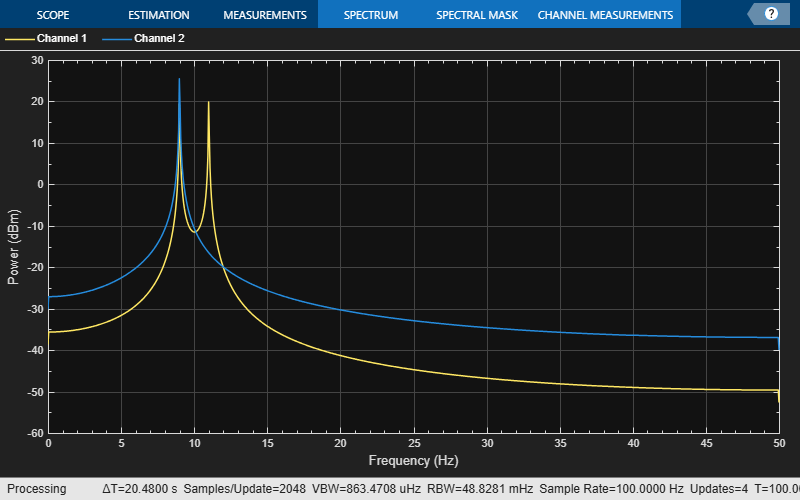# ammod

Amplitude modulation

## Syntax

``y = ammod(x,Fc,Fs)``
``y = ammod(x,Fc,Fs,ini_phase)``
``y = ammod(x,Fc,Fs,ini_phase,carramp)``

## Description

example

````y = ammod(x,Fc,Fs)` returns an amplitude modulated (AM) signal `y`, given the input message signal `x`, where the carrier signal has frequency `Fc`. The carrier signal and `x` have a sampling frequency `Fs`. The modulated signal has zero initial phase and zero carrier amplitude, so the result is suppressed-carrier modulation. NoteThe value of `Fs` must satisfy `Fs` ≥ 2`Fc`. ```
````y = ammod(x,Fc,Fs,ini_phase)` specifies the initial phase in the modulated signal `y` in radians.```
````y = ammod(x,Fc,Fs,ini_phase,carramp)` performs transmitted-carrier modulation instead of suppressed-carrier modulation where `carramp` is the carrier amplitude of the modulated signal.```

## Examples

collapse all

Set the sample rate to 100 Hz. Create a time vector 100 seconds long.

```fs = 100; t = (0:1/fs:100)';```

Set the carrier frequency to 10 Hz. Generate a sinusoidal signal.

```fc = 10; x = sin(2*pi*t);```

Modulate `x` using single- and double-sideband AM.

```ydouble = ammod(x,fc,fs); ysingle = ssbmod(x,fc,fs);```

Create a spectrum analyzer object to plot the spectra of the two signals. Plot the spectrum of the double-sideband signal.

```sa = dsp.SpectrumAnalyzer('SampleRate',fs, ... 'PlotAsTwoSidedSpectrum',false, ... 'YLimits',[-60 40]); sa(ydouble)```Plot the single-sideband spectrum.

`sa(ysingle)`## Input Arguments

collapse all

Input message signal, specified as a scalar, vector, matrix, or a 3-D array. Each element of `x` must be real.

Data Types: `single` | `double`

Carrier signal frequency in hertz (Hz), specified as a positive real scalar.

Data Types: `single` | `double`

Sampling frequency of carrier signal and input message signal in hertz (Hz), specified as a positive real scalar. To avoid aliasing, the value of `Fs` must satisfy `Fs` > 2(`Fc` + `BW`), where `BW` is the bandwidth of `x`.

Data Types: `single` | `double`

Initial phase of the modulated signal in radians, specified as a real scalar.

Data Types: `single` | `double`

Carrier amplitude of the modulated signal, specified as a real scalar.

Data Types: `single` | `double`

## Output Arguments

collapse all

Amplitude modulated signal, returned as a scalar, vector, matrix, or 3-D array.

## Version History

Introduced before R2006a